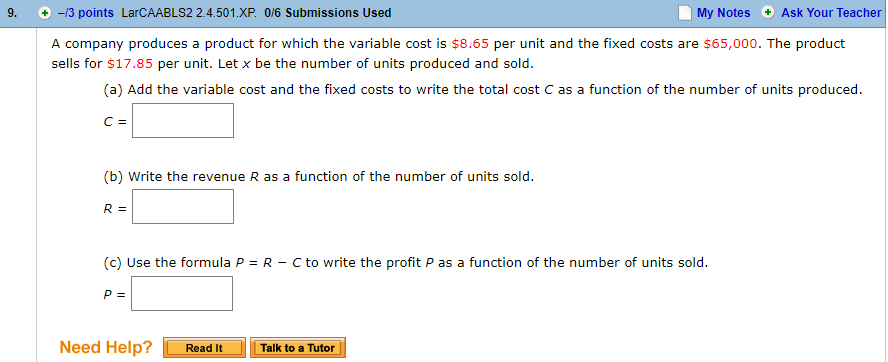# 9. -/3 points LarCAABLS2 2.4.501.xP. 0/6 Submissions Used My Notes Ask Your Teacher A company produces a product for which the variable cost is $8.65 per unit and the fixed costs are$65,000. The product sells for $17.85 per unit. Let x be the number of units produced and sold (a) Add the variable cost and the fixed costs to write the total cost C as a function of the number of units produced (b) Write the revenue R as a function of the number of units sold (c) Use the formula P R Cto write the profit P as a function of the number of units sold. Need Help?Read It Talk to a Tutor Questionhelp_outlineImage Transcriptionclose9. -/3 points LarCAABLS2 2.4.501.xP. 0/6 Submissions Used My Notes Ask Your Teacher A company produces a product for which the variable cost is$8.65 per unit and the fixed costs are $65,000. The product sells for$17.85 per unit. Let x be the number of units produced and sold (a) Add the variable cost and the fixed costs to write the total cost C as a function of the number of units produced (b) Write the revenue R as a function of the number of units sold (c) Use the formula P R Cto write the profit P as a function of the number of units sold. Need Help?Read It Talk to a Tutor fullscreen

### Want to see the step-by-step answer?